# Python Lambda Functions

## Python Programming Training Certification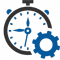Flexible Hours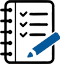100 Assignments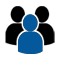Instructor Led online Training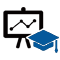50 LMS Access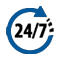24X7 Support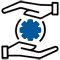100% Skill Level

### Enquire Now

4.9 out of 1000+ Ratings
Best Python Institute for Learning Python Course & Training, Live Project Training in Python with Django, Data Science and AI, Interview Assistance, Expert Coaching Trainers. Python Certification & Interview Assistance! Get free demo now!

### Course Overview

Python is one of the world’s top programming languages used today and Python training has become the most popular training across individuals. Training Basket’s Python Training & Certification course covers basic and advanced Python concepts and how to apply them in real-world applications.Python is a flexible and powerful open-source language that is easy to learn and consists of powerful libraries for data analysis and manipulation. Our Python training course content is curated by experts as per the standard Industry curriculum. The curriculum, coding challenges and real-life problems cover data operations in Python, strings, conditional statements, error handling, shell scripting, web scraping and the commonly used Python web framework Django. Take this Python training and certification course and become job-ready now.### Python Lambda Functions

Python Lambda function is known as the anonymous function that is defined without a name. Python allows us to not declare the function in the standard manner, i.e., by using the def keyword. Rather, the anonymous functions are declared by using the lambda keyword. However, Lambda functions can accept any number of arguments, but they can return only one value in the form of expression.

The anonymous function contains a small piece of code. It simulates inline functions of C and C++, but it is not exactly an inline function.

The syntax to define an anonymous function is given below.

Syntax

• lambda arguments: expression

It can accept any number of arguments and has only one expression. It is useful when the function objects are required.

Consider the following example of the lambda function.

Example 1

• # a is an argument and a+10 is an expression which got evaluated and returned.
x = lambda a:a+10
# Here we are printing the function object
print(x)
print(“sum = “,x(20))

Output:

• <function <lambda> at 0x0000019E285D16A8>
sum = 30

In the above example, we have defined the lambda a: a+10 anonymous function where a is an argument and a+10 is an expression. The given expression gets evaluated and returned the result. The above lambda function is same as the normal function.

• def x(a):
return a+10
print(sum = x(10))

Example 2

Multiple arguments to Lambda function

• # a and b are the arguments and a*b is the expression which gets evaluated and returned.
x = lambda a,b: a*b
print(“mul = “, x(20,10))
<p><strong>Output:</strong></p>
<div class=”codeblock”><pre>
mul = 200
</pre></div>
<h2 class=”h2″>Why use lambda function?</h2>
<p>The main role of the lambda function is better described in the scenarios when we use them anonymously inside another function. In Python, the lambda function can be used as an argument to the <strong>higher-order functions</strong> which accepts other functions as arguments.</p>
<p>Consider the following example:</p>
<strong>Example 1:</strong>
<div class=”codeblock”><textarea name=”code” class=”python”>
#the function table(n) prints the table of n
def table(n):
return lambda a:a*n # a will contain the iteration variable i and a multiple of n is returned at each function call
n = int(input(“Enter the number:”))
b = table(n) #the entered number is passed into the function table. b will contain a lambda function which is called again and again with the iteration variable i
for i in range(1,11):
print(n,”X”,i,”=”,b(i)) #the lambda function b is called with the iteration variable i

Output:

• Enter the number:10
10 X 1 = 10
10 X 2 = 20
10 X 3 = 30
10 X 4 = 40
10 X 5 = 50
10 X 6 = 60
10 X 7 = 70
10 X 8 = 80
10 X 9 = 90
10 X 10 = 100

The lambda function is commonly used with Python built-in functions filter() function and map() function.

Use lambda function with filter()

The Python built-in filter() function accepts a function and a list as an argument. It provides an effective way to filter out all elements of the sequence. It returns the new sequence in which the function evaluates to True.

Consider the following example where we filter out the only odd number from the given list.

• #program to filter out the tuple which contains odd numbers
lst = (10,22,37,41,100,123,29)
oddlist = tuple(filter(lambda x:(x%3 == 0),lst)) # the tuple contains all the items of the tuple for which the lambda function evaluates to true
print(oddlist)

Output:

• (37, 41, 123, 29)

Using lambda function with map()

The map() function in Python accepts a function and a list. It gives a new list which contains all modified items returned by the function for each item.

Consider the following example of map() function.

• #program to filter out the list which contains odd numbers
lst = (10,20,30,40,50,60)
square_list = list(map(lambda x:x**2,lst)) # the tuple contains all the items of the list for which the lambda function evaluates to true
print(square_tuple)

Output:

• (100, 400, 900, 1600, 2500, 3600)

### Recently Trained Students#### Jessica Biel

– Infosys

My instructor had sound Knowledge and used to puts a lot of effort that made the course as simple and easy as possible. I was aiming for with the help of the ZebLearn Online training imparted to me by this organization.#### Richard Harris

– ITC

I got my training from Zeblearn in the Python Certification Training, I would like to say that say he is one of the best trainers. He has not even trained me but also motivated me to explore more and the way he executed the project, in the end, was mind-blowing.

### Candidate’s Journey During Our Training Program#### Expert’s Advice & Selection of Module

Choosing the right type of module for the training is half the battle & Our Team of experts will help & guide you.#### Get Trained

Get Trained & Learn End to End Implementation from our Expert Trainer who are working on the same domain.#### Work on Projects

We Do make our student’s work on multiple case studies , scenario based tasks & projects in order to provide real-time exposure to them.#### Placements

We have a dedicated placement cell in order to provide placement assistance & relevant interviews to our candididates till selection